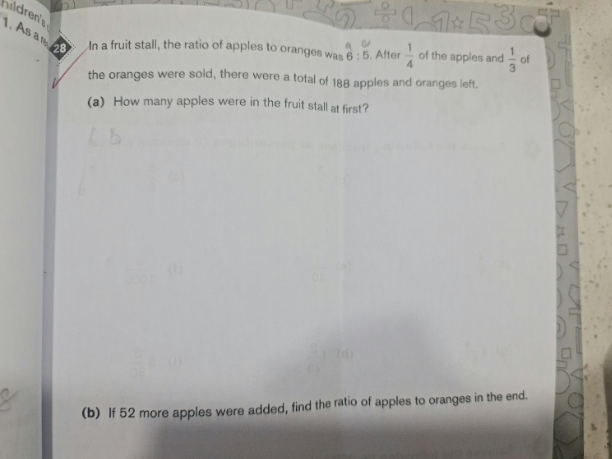# QuestionHi,

Need help. Thanks

Apples               Oranges

6u                       5u

Sold         1/4                    1/3

Left         3/4=9/12        2/3 = 8/12

(9/12)(6u) + (8/12)(5u) = 188

54u + 40U = 2256

u = 2256/94 = 24

(a)  Apples at first = 6u =6 x 24 = 144

(b)  Apples left = (3/4)(144) = 108    Add 52 => Apples = 108+52 = 160

Oranges left = 188-108 = 80

Hence final ratio of apples to oranges =  160 : 80 = 2 : 1

Thank you so much.

Thanks sushi88!  Here’s how I do it by equations:

(a)
A/O = 6/5
=> O = (5/6)A … (1)

(3/4)A + (2/3)O = 188
(3/2)(3/4)A + O =(3/2)188 = 282
=> O = 282 – (9/8)A … (2)

Substituting (1) into 2,
(5/6)A = 282 – (9/8)A
(5/6)A + (9/8)A = 282
((40+54)/48)A = 282
(94/48)A = 282
A = (48/94) 282 = 144 #

(b) Original O = (5/6)A = 120
Oranges left = 120 – (1/3)120 = 80
Now, apples become 144 – (1/4)144 + 52 = 160
Ratio A:O = 160 : 80 = 2 : 1 #

0 Replies 1 Like